## 1.1- What is face recognition?

Face verification（人脸验证） vs. face recognition（人脸识别）

• 人脸验证：一对一的问题，给定一张人脸图片和一个身份信息，验证图片和身份是否匹配
• 人脸识别：要比人脸验证困难，一对多问题。对一个K个人的数据库，给定一张图片，判断这张图片是K个人种的哪一个（或不是K中的任何一个）

## 1.3- Siamese Network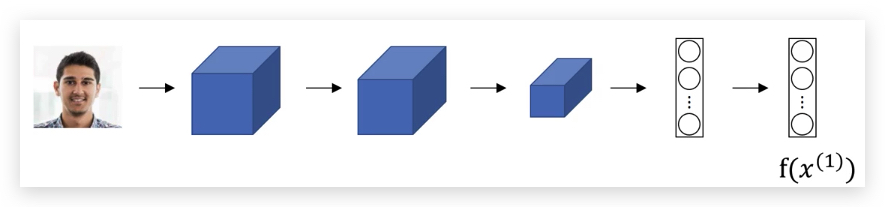$d(x^{(1)}, x^{(2)}) = ||f(x^{(1)}) - f(x^{(2)})||^2_2$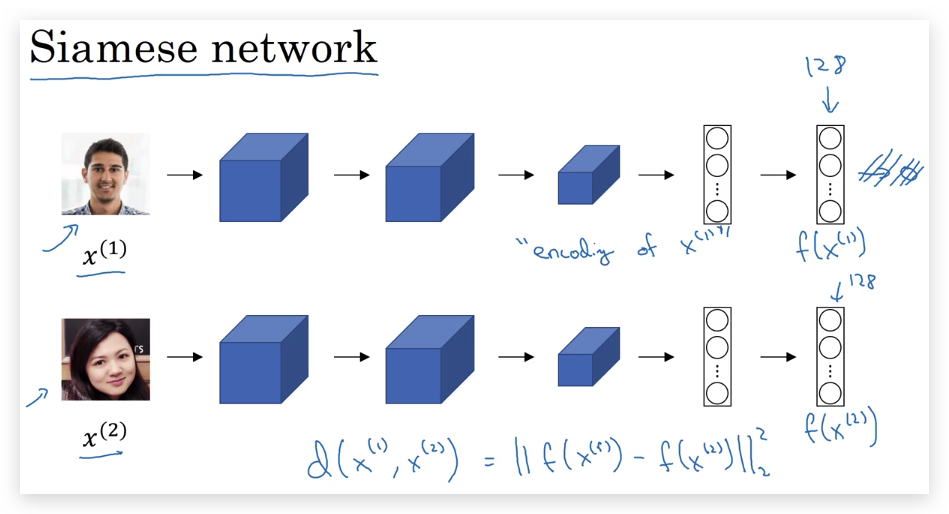• NN网络的参数决定了编码$$f(x^{(i)})$$
•  学习这样的参数，使得如果$$x^{(i)}$$和$$x^{(j)}$$表示同一个人，则$$f(x^{(i)}) - f(x^{(j)}) ^2_2$$的值非常小。如果表示不同的人，则$$f(x^{(i)}) - f(x^{(j)}) ^2_2$$非常大。

## 1.4- Triplet Loss

• Anchor
• Positive
• Negative

$(||f(x^{(A)}) - f(x^{(P)})||^2_2 \le ||f(x^{(A)}) - f(x^{(N)})||^2_2$

$||f(x^{(A)}) - f(x^{(P)})||^2_2 - ||f(x^{(A)}) - f(x^{(N)})||^2_2 \le 0$

$||f(x^{(A)}) - f(x^{(P)})||^2_2 - ||f(x^{(A)}) - f(x^{(N)})||^2_2 + \alpha \le 0$

$L(A,P,N) = max(||f(x^{(A)}) - f(x^{(P)})||^2_2 - ||f(x^{(A)}) - f(x^{(N)})||^2_2 + \alpha, 0)$

$J = \sum^m_{i=1}L(A^{(i)}, P^{(i)}, N^{(i)})$

## 1.5- Face Verification and Binary Classification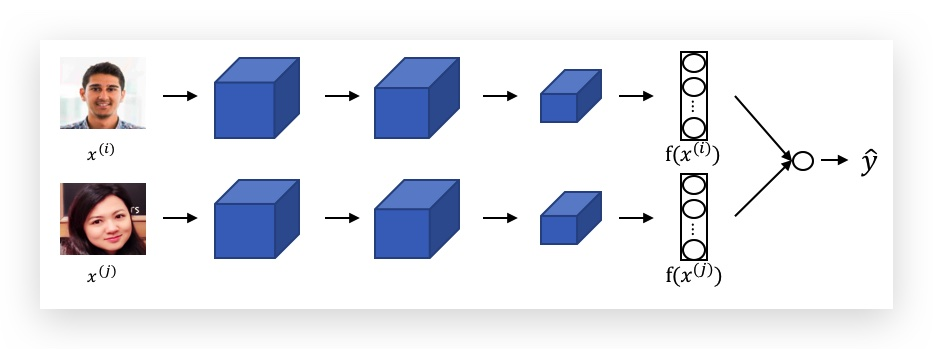$\hat y = \sigma (\sum^K_{k=1}w_k|f(x^{(i)})\_k - f(x^{(j)})\_k)| + b)$

$\hat y = \sigma (\sum^K_{k=1}w_k \frac{(f(x^{(i)})_k - f(x^{(j)})_k)^2}{f(x^{(i)})_k + f(x^{(j)})_k} + b)$

## 2.1- What is neural style transfer?

Neural Stlye Transfer，直观上就是将一种图片的风格应用到另一张内容图片上，比如：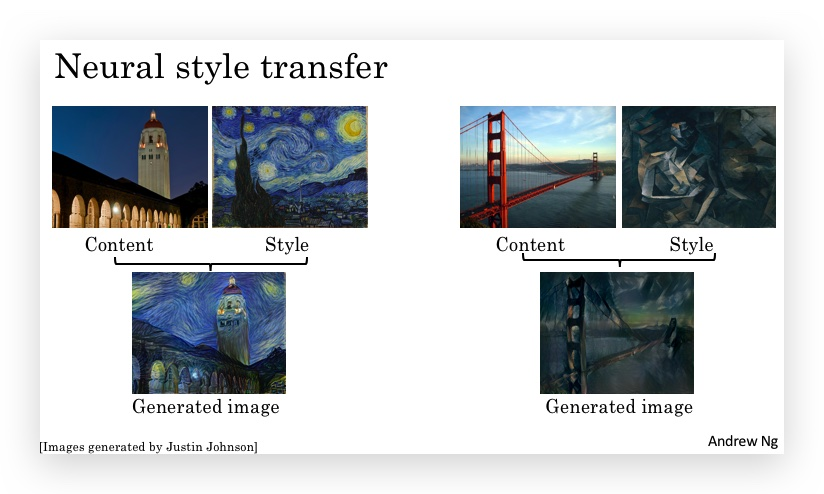## 2.2- What are deep ConvNets learning?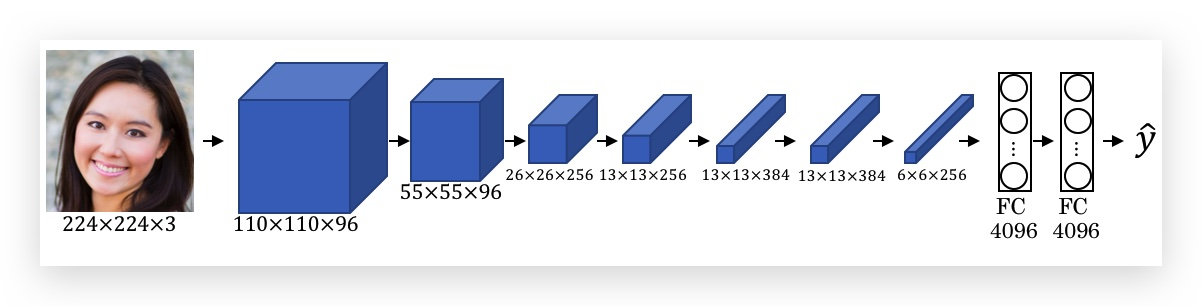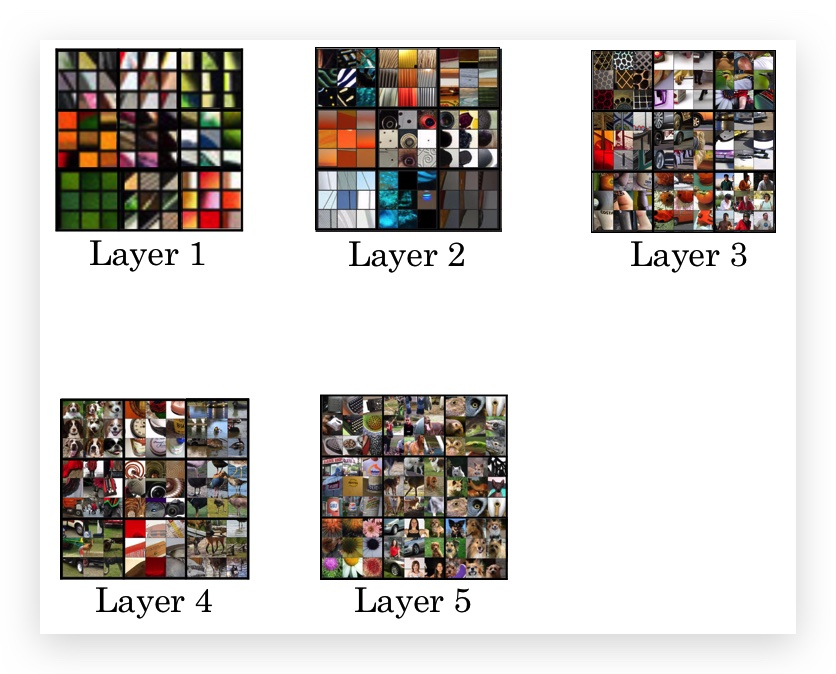• 浅层网络识别了图像的边缘、纹理、颜色的过渡等较简单的低层次特征；
• 深层的unit，其识别的区域较大，特性更具体，比如狗的脸、汽车的轮子等。
• 观察每个unit的9个色块，很容易发现具有相似的共性特性，即该unit识别的特征。

## 2.3- Cost Function

$J(G) = \alpha \cdot J_{content}(C, G) + \beta \cdot J_{style}(S, G)$

• 随机生成G的每个像素点。（即参数初始化）
• 使用Gradient Descent最小化Cost Function $$J(G)$$，在这个过程中G会慢慢的生成，就像这样：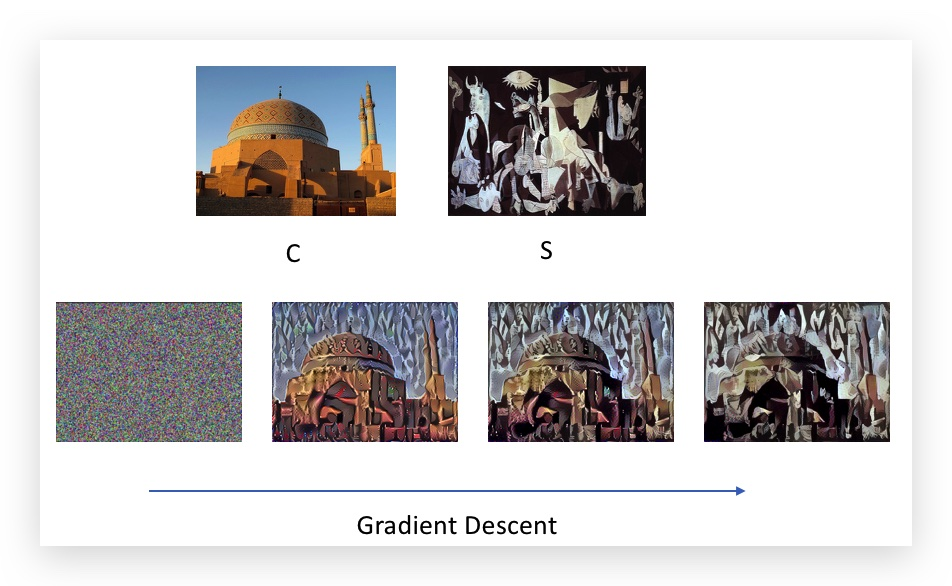## 2.4- Content Cost Function

• 使用一个训练好的ConvNet，如VGG网络。（这个ConvNet的训练不属于Style Transfer的范围）
• 使用ConvNet的某一个隐藏层$$l$$计算Content Cost。隐藏层$$l$$的选择，通常是网络中处于中间的隐藏层。如果太浅，则隐藏层和原始图片看起来差不多，如果太深，则太过于具体。
• 图片C和G的Cost Function可表示为： $$J_{content}(C, G) = \frac{1}{2}||(a^{(C)[l]} - a^{(G)[l]})||^2$$ 其中，$$a^{(C)[l]}$$和$$a^{(G)[l]}$$分别为C和G在$$l$$层的激活函数。

## 2.5- Style Cost Function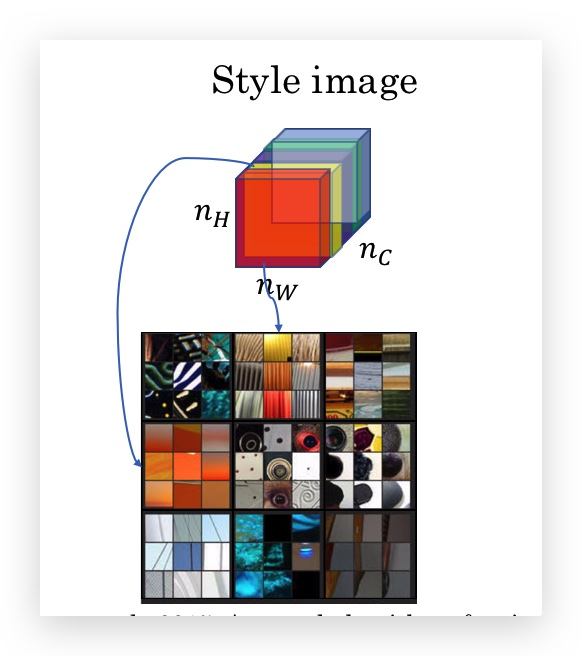channel的相关性，表名了某些特征多大机会同时出现或不同时出现。style就是某些特征的混杂。

1. 首先定义两个符号：
• 用$$a^{[l]}_{i,j,k}$$ 表示第$$l$$层在volume中处于$$(i,j,k)$$位置的激活函数。其中$$i$$是width（即在$$n^{[l]}_W$$的维度上），$$j$$是Height（即在即在$$n^{[l]}_H$$的维度上），而$$k$$是channel（即在$$n^{[l]}_C$$的维度上）。
• 用$$G^{[l]}$$表示style matrix，实际上就是$$l$$层所有channel组成的相关矩阵，所以其维度是$$n^{[l]}_C \times n^{[l]}_C$$。而$$G^{[l]}_{kk’}$$即第$$(k,k’)$$个元素，代表第$$k$$个channel和第$$k’$$个channel的相关性。style matrix之所以用大写字母G表示style matrix，是因为在数学上，它称之为gram matrix
2. Style matrix $$G^{[l]}_{kk’}$$的计算如下：

$G^{[l]}\_{kk'} = \sum^{n^{[l]}\_H}\_{i=1} \sum^{n^{[l]}\_W}\_{j=1} a^{[l]}\_{ijk} a^{[l]}\_{ijk'}$

直观的来说，就是第$$k$$个channel和第$$k’$$个channel这两个矩阵的元素级乘积的和。可以理解成向量的内积，而内积反应的就是相关性。

对于style matrix，我们需要分别在图片S和图片G上计算，因此再增加一个上标，分别表示S和G的style matrix的计算：

$G^{[l]$$S)}\_{kk'} = \sum^{n^{[l]}\_H}\_{i=1} \sum^{n^{[l]}\_W}\_{j=1} a^{[l]\(S)}\_{ijk} a^{[l]\(S)}\_{ijk'}$ $G^{[l]\(G)}\_{kk'} = \sum^{n^{[l]}\_H}\_{i=1} \sum^{n^{[l]}\_W}\_{j=1} a^{[l]\(G)}\_{ijk} a^{[l]\(G)}\_{ijk'}$ 3. 有了S和G的style matrix，就可以定义style的cost function了，即两个style的差异： $J^{[l]}\_{style}(S, G) = \frac{1}{(2n^{[l]}\_Hn^{[l]}\_Wn^{[l]}\_C)^2} || G^{[l]\(S)} - G^{[l]\(G)}||^2_F$ 即两个style matrix元素级相减后，再将元素做平方和（下标F表示Frobenius norm，在之前的课程有提到：L2 Regularization）展开后即： $J^{[l]}\_{style}(S, G) = \frac{1}{(2n^{[l]}\_Hn^{[l]}\_Wn^{[l]}\_C)^2} \sum_k^{n^{[l]}\_C} \sum_{k'}^{n^{[l]}\_C}(G^{[l]\(S)}\_{kk'} - G^{[l]\(G)}\_{kk'})^2$ 1. \(J^{[l]}_{style}(S, G)$$只是第$$l$$层的cost function，所有层的cost function之和组成了全部的cost function：
$J_{style}(S, G) = \sum_l^L \lambda^{[l]} J^{[l]}\_{style}(S, G)$

## 2.6- 1D and 3D Generalizations

1. 1D卷积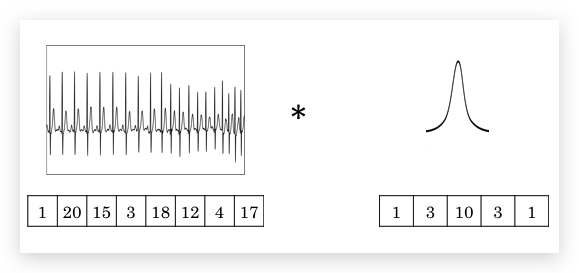1D卷积网络，可以应用于1D数据，但事实上更常用的是递归神经网网络（RNN, Recurrent Neural Network）。

1. 3D卷积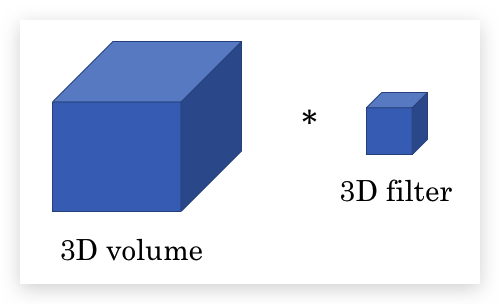-------------------------